### Python Lab Tutorials: Lab 3

Create a procedure whose inputs are the function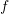, the value of, the value of, the maximum number of iterations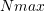, the value of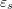, and the value of the flag = 1 or 2.
The procedure should do the following:

• If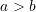, the procedure should give an error: “Please select a < b”
• If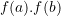is not less than zero, the procedure should give an error: “Please select appropriate values a and b such that f(a)*f(b)<0″
• If flag is not equal to 1 or 2, the procedure should output: “Please select appropriate values (1 or 2) for flag”
• If flag = 1, then the procedure should implement the bisection method to output the table of [iteration, a,b,x_i,f(a),f(b),f(xi),e_r]
• If flag = 2, then the procedure should implement the false position method to output the table of [iteration, a,b,x_i,f(a),f(b),f(xi),e_r]

Show that your procedure works by trying it on examples that would generate errors. Then, pick one of the problems in the bracketing methods section and show that your procedure works for both methods.